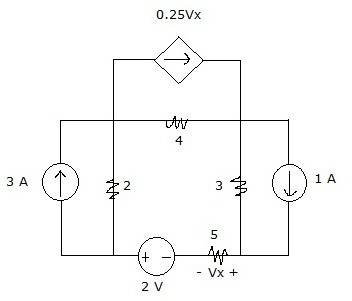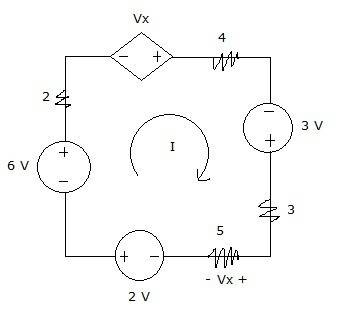# Circuit Analysis - Source Transformation

• Engineering

## Homework Statement

Find Vx using source transformationV=IR
KVL

## The Attempt at a Solution

After doing source transformation on the three pairs of current sources and resistors...KVL:
-6+2I-Vx+4I-3+3I+5I-2=0
14I-Vx-11=0
14I-5I-11=0
1I=11
I=1.222 A

Vx=-5I=-5(1.222)
Vx=-6.11 V

Did I do the source transformation right? Also, I'm confused with the value of Vx. Is it -5I or just 5I? Because in our class, our convention is that when a current source is transformed into a voltage source, the part where the arrow head in the current source becomes the positive terminal for the voltage source. But since we're only dealing with a resistor here, I just followed our convention for the KVL (the voltage across 5 ohms is positive since the mesh current enters the positive terminal)

Last edited: